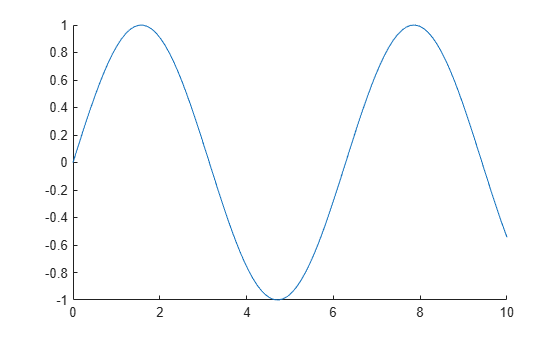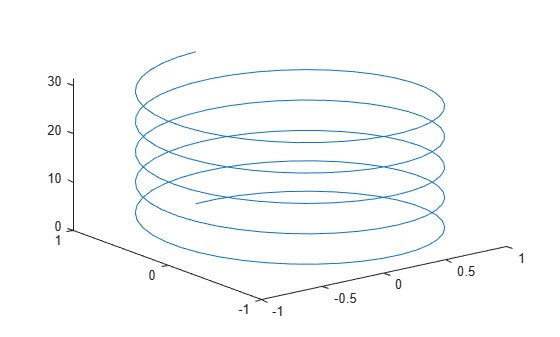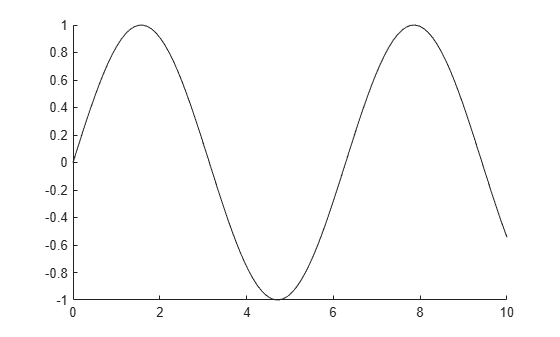# line

Create primitive line

## Syntax

``line(x,y)``
``line(x,y,z)``
``line``
``line(___,Name,Value)``
``line(ax,___)``
``pl = line(___)``

## Description

example

````line(x,y)` plots a line in the current axes using the data in vectors `x` and `y`. If either `x` or `y`, or both are matrices, then `line` draws multiple lines. Unlike the `plot` function, `line` adds the line to the current axes without deleting other graphics objects or resetting axes properties. ```

example

````line(x,y,z)` plots a line in three-dimensional coordinates.```
````line` draws a line from the point `(0,0)` to `(1,1)` with the default property settings.```

example

````line(___,Name,Value)` modifies the appearance of the line using one or more name-value argument pairs. For example, `'LineWidth',3` sets the line width to `3` points. Specify name-value pairs after all other input arguments. If you specify the data using name-value pairs, for example `line('XData','x','YData','y')`, then you must specify vector data.```
````line(ax,___)` creates the line in the Cartesian, polar, or geographic axes specified by `ax` instead of in the current axes (`gca`). Specify `ax` as the first input argument.```

example

````pl = line(___)` returns all primitive `Line` objects created. Use `pl` to modify properties of a specific `Line` object after it is created. For a list, see Line Properties.```

## Examples

collapse all

Create `x` and `y` as vectors. Then plot `y` versus `x`.

```x = linspace(0,10); y = sin(x); line(x,y)```Plot two lines by specifying `x` and `y` as matrices. Use `line` to plot columns of `y` versus columns of `x` as separate lines.

```x = linspace(0,10)'; y = [sin(x) cos(x)]; line(x,y)```Plot a line in 3-D coordinates by specifying x, y, and z values. Change the axes to a 3-D view using `view(3)`.

```t = linspace(0,10*pi,200); x = sin(t); y = cos(t); z = t; line(x,y,z) view(3)```Create `x` and `y` as vectors. Then plot `y` versus `x` using the low-level version of the `line` function.

```x = linspace(0,10); y = sin(x); line('XData',x,'YData',y)```Draw a red, dashed line between the points (1,2) and (9,12). Set the `Color` and `LineStyle` properties as name-value pairs.

```x = [1 9]; y = [2 12]; line(x,y,'Color','red','LineStyle','--')```First, draw a line from the point (3,15) to (2,12) and return the `Line` object. Then change the line to a green, dashed line. Use dot notation to set properties.

```x = [3 2]; y = [15 12]; pl = line(x,y);``````pl.Color = 'green'; pl.LineStyle = '--';```## Input Arguments

collapse all

First coordinate, specified as a vector or a matrix. Matrix inputs are supported for Cartesian axes only.

The interpretation of the first coordinate depends on the type of axes. For Cartesian axes, the first coordinate is x-axis position in data units.

• If `x` and `y` are both vectors with the same length, then `line` plots a single line.

• If `x` and `y` are matrices with the same size, then `line` plots multiple lines. The function plots columns of `y` versus `x`.

• If one of `x` or `y` is a vector and the other is a matrix, then `line` plots multiple lines. The length of the vector must equal one of the matrix dimensions:

• If the vector length equals the number of matrix rows, then `line` plots each matrix column versus the vector.

• If the vector length equals the number of matrix columns, then `line` plots each matrix row versus the vector.

• If the matrix is square, then `line` plots each column versus the vector.

For polar axes, the first coordinate is the polar angle θ in radians. For geographic axes, the first coordinate is latitude in degrees. To plot lines in these types of axes, `x` and `y` must be the same size.

Example: `x = linspace(0,10,25)`

Data Types: `single` | `double` | `int8` | `int16` | `int32` | `int64` | `uint8` | `uint16` | `uint32` | `uint64` | `categorical` | `datetime` | `duration`

Second coordinate, specified as a vector or a matrix. Matrix inputs are supported for Cartesian axes only.

The interpretation of the second coordinate depends on the type of axes. For Cartesian axes, the second coordinate is y-axis position in data units.

• If `x` and `y` are both vectors with the same length, then `line` plots a single line.

• If `x` and `y` are matrices with the same size, then `line` plots multiple lines. The function plots columns of `y` versus `x`.

• If one of `x` or `y` is a vector and the other is a matrix, then `line` plots multiple lines. The length of the vector must equal one of the matrix dimensions:

• If the vector length equals the number of matrix rows, then `line` plots each matrix column versus the vector.

• If the vector length equals the number of matrix columns, then `line` plots each matrix row versus the vector.

• If the matrix is square, then `line` plots each column versus the vector.

For polar axes, the second coordinate is the radius in data units. For geographic axes, the second coordinate is longitude in degrees. To plot lines in these types of axes, `x` and `y` must be the same size.

Example: `y = sin(x)`

Data Types: `single` | `double` | `int8` | `int16` | `int32` | `int64` | `uint8` | `uint16` | `uint32` | `uint64` | `categorical` | `datetime` | `duration`

Third coordinate, specified as a vector or a matrix. Matrix inputs are supported for Cartesian axes only.

The interpretation of the third coordinate depends on the type of axes. For Cartesian axes, the third coordinate is z-axis position in data units.

• If `x`, `y`, and `z` are all vectors with the same length, then `line` plots a single 3-D line.

• If `x`, `y`, and `z` are all matrices with the same size, then `line` plots multiple 3-D lines using the matrix columns.

• If one or two of `x`, `y`, and `z` is a vector and the others are matrices of the same size, then `line` plots multiple 3-D lines. The length of the vector must equal one of the matrix dimensions.

For polar and geographic axes, the third coordinate affects the layering of 2-D lines on the axes. To use the third coordinate in these types of axes, `x`, `y`, and `z` must be the same size.

Example: `z = sin(x) + cos(y)`

Data Types: `single` | `double` | `int8` | `int16` | `int32` | `int64` | `uint8` | `uint16` | `uint32` | `uint64` | `categorical` | `datetime` | `duration`

Target axes, specified as an `Axes` object, a `PolarAxes` object, or a `GeographicAxes` object. If you do not specify the axes, then the `line` function plots in the current axes.

### Name-Value Pair Arguments

Specify optional comma-separated pairs of `Name,Value` arguments. `Name` is the argument name and `Value` is the corresponding value. `Name` must appear inside quotes. You can specify several name and value pair arguments in any order as `Name1,Value1,...,NameN,ValueN`.

Example: `line(x,y,'Color','red','LineWidth',3)` creates a red line that is 3 points wide.

The properties listed here are only a subset. For a full list, see Line Properties.

Line color, specified as an RGB triplet, a hexadecimal color code, a color name, or a short name.

For a custom color, specify an RGB triplet or a hexadecimal color code.

• An RGB triplet is a three-element row vector whose elements specify the intensities of the red, green, and blue components of the color. The intensities must be in the range `[0,1]`; for example, ```[0.4 0.6 0.7]```.

• A hexadecimal color code is a character vector or a string scalar that starts with a hash symbol (`#`) followed by three or six hexadecimal digits, which can range from `0` to `F`. The values are not case sensitive. Thus, the color codes `'#FF8800'`, `'#ff8800'`, `'#F80'`, and `'#f80'` are equivalent.

Alternatively, you can specify some common colors by name. This table lists the named color options, the equivalent RGB triplets, and hexadecimal color codes.

Color NameShort NameRGB TripletHexadecimal Color CodeAppearance
`'red'``'r'``[1 0 0]``'#FF0000'``'green'``'g'``[0 1 0]``'#00FF00'``'blue'``'b'``[0 0 1]``'#0000FF'``'cyan'` `'c'``[0 1 1]``'#00FFFF'``'magenta'``'m'``[1 0 1]``'#FF00FF'``'yellow'``'y'``[1 1 0]``'#FFFF00'``'black'``'k'``[0 0 0]``'#000000'``'white'``'w'``[1 1 1]``'#FFFFFF'``'none'`Not applicableNot applicableNot applicableNo color

Here are the RGB triplets and hexadecimal color codes for the default colors MATLAB® uses in many types of plots.

`[0 0.4470 0.7410]``'#0072BD'``[0.8500 0.3250 0.0980]``'#D95319'``[0.9290 0.6940 0.1250]``'#EDB120'``[0.4940 0.1840 0.5560]``'#7E2F8E'``[0.4660 0.6740 0.1880]``'#77AC30'``[0.3010 0.7450 0.9330]``'#4DBEEE'``[0.6350 0.0780 0.1840]``'#A2142F'`Example: `line(x,y,'Color','blue')`

Example: `line(x,y,'Color',[0.5 0.5 1])`

Example: `line(x,y,'Color','#D9A2E9')`

Line style, specified as one of the options listed in this table.

Line StyleDescriptionResulting Line
`'-'`Solid line`'--'`Dashed line`':'`Dotted line`'-.'`Dash-dotted line`'none'`No lineNo line

Line width, specified as a positive value in points, where 1 point = 1/72 of an inch. If the line has markers, then the line width also affects the marker edges.

The line width cannot be thinner than the width of a pixel. If you set the line width to a value that is less than the width of a pixel on your system, the line displays as one pixel wide.

Marker symbol, specified as one of the values listed in this table. By default, the object does not display markers. Specifying a marker symbol adds markers at each data point or vertex.

ValueDescription
`'o'`Circle
`'+'`Plus sign
`'*'`Asterisk
`'.'`Point
`'x'`Cross
`'_'`Horizontal line
`'|'`Vertical line
`'square'` or `'s'`Square
`'diamond'` or `'d'`Diamond
`'^'`Upward-pointing triangle
`'v'`Downward-pointing triangle
`'>'`Right-pointing triangle
`'<'`Left-pointing triangle
`'pentagram'` or `'p'`Five-pointed star (pentagram)
`'hexagram'` or `'h'`Six-pointed star (hexagram)
`'none'`No markers

Marker size, specified as a positive value in points, where 1 point = 1/72 of an inch.

## Output Arguments

collapse all

Primitive line object. Use `pl` to query or modify properties of the line after it is created. For a list, see Line Properties.

## Tips

• Unlike the `plot` function, the `line` function does not call `newplot` before plotting and does not respect the value of the `NextPlot` property for the figure or axes. It simply adds the line to the current axes without deleting other graphics objects or resetting axes properties. However, some axes properties, such as the axis limits, can update to accommodate the line.

## Extended Capabilities

### Topics

Introduced before R2006a